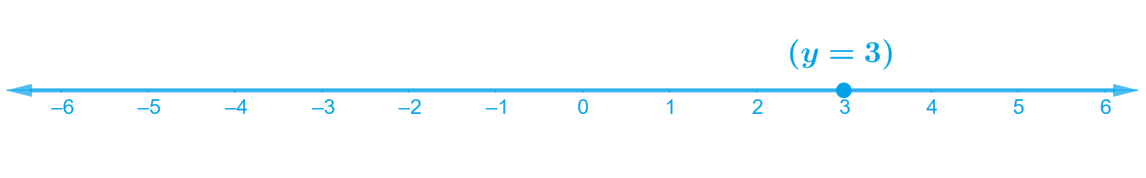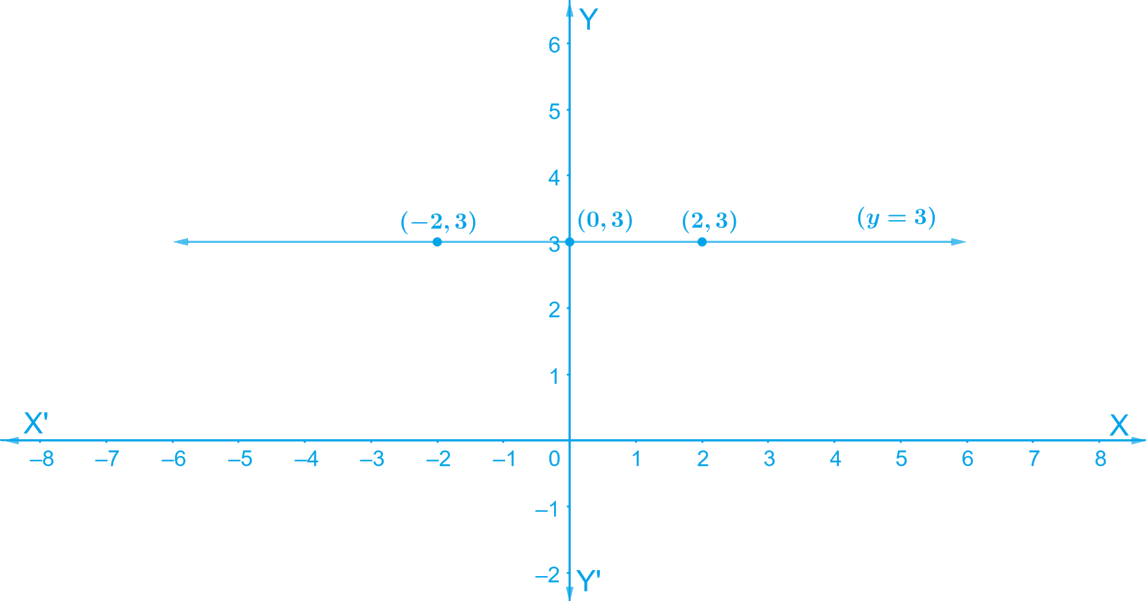In the verge of coronavirus pandemic, we are providing FREE access to our entire Online Curriculum to ensure Learning Doesn't STOP!

# Ex.4.4 Q1 Linear Equations in Two Variables Solution - NCERT Maths Class 9

Go back to  'Ex.4.4'

## Question

Give the geometric representation of $$y = 3$$ as an equation

(i) in one variable

(ii) in two variables

Video Solution
Linear Equations In Two Variables
Ex 4.4 | Question 1

## Text Solution

#### Steps:

(i) Given,

Considering $$y = 3$$ is the equation in one variable

The representation of the solution on the number when $$y = 3$$ is treated as an equation in one variable

In one variable, $$y = 3$$ represents a point as shown in following figure.(ii) Given:

Considering $$y = 3$$ is the equation in two variables

We know that $$y = 3$$ can be written as $$0.x + y = 0$$.

In two variables, $$y = 3$$ represents a straight line passing through point $$(0, 3)$$ and parallel to $$x$$-axis. It is a collection of all the points on the plane, having their $$y$$-coordinate as $$3.$$

Hence,

• When, $$x = 0$$, we get $$y = 3$$;
• When $$x = 2$$, we get $$y = 3$$;
• When $$x = -2$$, we get $$y = 3$$ are the solutions for the equations.

Plotting the points $$(0, 3) (2, 3)$$ and $$(–2, 3)$$ and on joining them we get the graph $$AB$$ as a line parallel to $$x$$-axis at a distance of $$3\,\rm units$$ above it

The graphical representation is shown below:Video Solution
Linear Equations In Two Variables
Ex 4.4 | Question 1

Learn from the best math teachers and top your exams

• Live one on one classroom and doubt clearing
• Practice worksheets in and after class for conceptual clarity
• Personalized curriculum to keep up with school